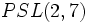# Direct product of SL(2,5) and PSL(3,2)

View a complete list of particular groups (this is a very huge list!)[SHOW MORE]

## Definition

This group is defined as the external direct product of the following two groups:

1. The group$SL(2,5)$, i.e., special linear group:SL(2,5) (order 120), which is also the double cover of alternating group:A5.
2. The group$PSL(3,2)$, i.e., projective special linear group:PSL(3,2) (order 168), which is also the projective special linear group of degree two over field:F7, i.e., the group$PSL(2,7)$.

## Arithmetic functions

Want to compare and contrast arithmetic function values with other groups of the same order? Check out groups of order 20160#Arithmetic functions
Function Value Similar groups Explanation
order (number of elements, equivalently, cardinality or size of underlying set) 20160 groups with same order order of direct product is product of orders, so the order is$120 \times 168 = 20160$

## Group properties

Property Satisfied? Explanation
abelian group No
nilpotent group No
solvable group No
simple group, simple non-abelian group No
quasisimple group No
directly indecomposable group No
perfect group Yes

## GAP implementation

Description Functions used
DirectProduct(SL(2,5),PSL(3,2)) DirectProduct, SL, PSL
PerfectGroup(20160,2) PerfectGroup